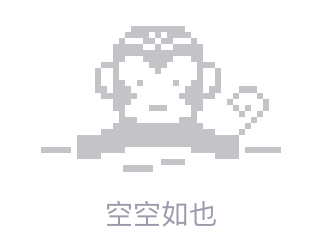# 未捕获的PDOException：SQLSTATE [HY093]：参数号无效：参数未定义[重复]

Uncaught PDOException: SQLSTATE[HY093]: Invalid parameter number: parameter was not defined on line 73.

What is this error ?

``````\$stmtExec = \$stmt->execute(); // the line 73
``````

Employee.php

``````public function update(\$fields,\$id){
\$st = "";
\$counter = 1;
\$total_fields = count(\$fields);
foreach (\$fields as \$key => \$value) {
if (\$counter === \$total_fields) {
\$set = "\$key = :".\$key;
\$st = \$st.\$set;
} else{
\$set = "\$key = :".\$key.",";
\$st = \$st.\$set;
\$counter++;
}
}

\$sql = "";
\$sql.= "UPDATE info SET".\$st;
\$sql.= "WHERE id =".\$id;

\$stmt = \$this->connect()->prepare(\$sql);

foreach (\$fields as \$key => \$value) {
\$stmt->bindValue(':'.\$key, \$value);
}

\$stmtExec = \$stmt->execute(); // here is the line with the error

if (\$stmtExec) {
}
}
``````
``````</div>
``````2018/07/05 08:34
• php
• mysql
• pdo
• 点赞
• 收藏
• 回答
私信

#### 1个回复